# Cube walls

Find the volume and the surface area of the cube if the area of one of its walls is 40 cm2.

Result

V =  252.982 cm3
S =  240 cm2

#### Solution:

$S_{ 1 } = 40 \ cm^2 \ \\ a = \sqrt{ S_{ 1 } } = \sqrt{ 40 } = 2 \ \sqrt{ 10 } \ cm \doteq 6.3246 \ cm \ \\ \ \\ V = a^3 = 6.3246^3 = 80 \ \sqrt{ 10 } \doteq 252.9822 = 252.982 \ cm^3$
$S = 6 \cdot \ S_{ 1 } = 6 \cdot \ 40 = 240 = 240 \ cm^2$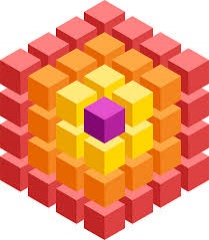Our examples were largely sent or created by pupils and students themselves. Therefore, we would be pleased if you could send us any errors you found, spelling mistakes, or rephasing the example. Thank you!

Leave us a comment of this math problem and its solution (i.e. if it is still somewhat unclear...):Be the first to comment!Tips to related online calculators
Tip: Our volume units converter will help you with the conversion of volume units.

## Next similar math problems:

1. Length of the edgeFind the length of the edge of a cube that has a cm2 surface and a volume in cm3 expressed by the same number.
2. A plasticineJožko modeled from plasticine. He used 27g of plasticine to model a 3 cm long cube. How many grams of plasticine will it need to mold cubes with an edge of 6cm?
3. Cube constructionA 2×2×2 cube is to be constructed using 4 white and 4 black unit cube. How many different cubes can be constructed in this way? ( Two cubes are not different if one can be obtained by rotating the other. )
4. Uboid volumeCalculate the cuboid volume if the walls are 30cm², 35cm², 42cm²
5. Octagonal pyramidFind the volume of a regular octagonal pyramid with height v = 100 and the angle of the side edge with the plane of the base is α = 60°.
6. Axial section of the coneThe axial section of the cone is an isosceles triangle in which the ratio of cone diameter to cone side is 2: 3. Calculate its volume if you know its area is 314 cm square.
7. Cone sideCalculate the volume and area of the cone whose height is 10 cm and the axial section of the cone has an angle of 30 degrees between height and the cone side.
8. Cylinder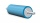The 1.8m cylinder contains 2000 liters of water. What area (in dm2) of this container is the water?
9. Tetrahedral pyramidDetermine the surface of a regular tetrahedral pyramid when its volume is V = 120 and the angle of the sidewall with the base plane is α = 42° 30´.
10. Conical bottleWhen a conical bottle rests on its flat base, the water in the bottle is 8 cm from it vertex. When the same conical bottle is turned upside down, the water level is 2 cm from its base. What is the height of the bottle?
11. A square base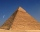A solid right pyramid has a square base. The length of the base edge is 4 centimeters and the height of the pyramid is 3 centimeters. What is the volume of the pyramid?
12. Swimming poolThe swimming pool has the shape of a block with dimensions of 70dm, 25m, 200cm. How many hl of water can fit into the pool?
13. A filterIt is a pool with a volume of 3500 liters. The filter filters at 4m cubic per hour. How many minutes would it filter the entire pool?
14. MilkThere were 22 liters of milk in three containers. There was 6 liters more in the first container than in the second. After pouring 5 liters from the first container into the third container, the same quantity of milk is in the second and third container.
15. Tributaries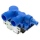The pool can be filled with two different tributaries. The first inflow would fill the pool in 18 hours, both in 6 hours. How many hours would the pool filled with a second inflow?
16. Pouring alcohol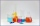100 liters of alcohol has 70% How many liters of water need to be added to have 60% alcohol?
17. The wellbore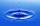The wellbore has a tributary of 2 m3 per hour. When there is no tapping, there are a stable 28 liters of water in the well. The pump suction basket is at the bottom of the well. At 14.00, the water was pumped out at a rate of 0.5 liters of water every se